﻿ 120-Degree Conduction DC Motor (with Switches)Examples | Product | Murata Software Co., Ltd.Example10120-Degree Conduction DC Motor (with Switches)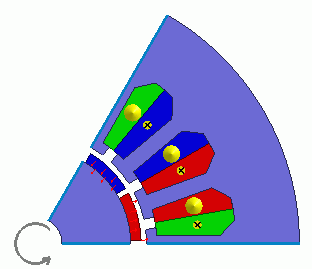General

• The basic characteristics of a 120-degree conduction DC brushless motor are analyzed.

• The torque, magnetic flux density distribution, iron loss, are solved.

• The analysis is coupled with the external circuit.

• The homogenizing method is applied to simulate the layer structure of the electromagnetic plates of steel for the core.

• Unless specified in the list below, the default conditions will be applied.

• In this example, a quarter period symmetric model is used for faster calculation.

Show Results

 Item Setting Analysis Space 2D Thickness in Depth Direction 10[mm] Unit mm Solver Magnetic Analysis [Luvens] Analysis Type Transient Analysis Options Select External Circuit Coupling. Select Rotating machinery.   [Partial Model (Symmetric Model) Setting] Select Partial Model. Division number: 6 [Conversion of external circuit I/O values] Select Convert Number of series: 1 Number of parallels: 6 Select Convert the result to the full model for output

The Rotating Machinery tab is set as follows.

 Tab Setting Item Setting Rotating Machinery Rotational Movement Select Constant Velocity The Number of Rotations: 5000[r/min] Rotor’s Initial Rotation Position: 0[deg] The Number of Slide Mesh Divisions Circumferential Division Angle: 1.0[deg] Rotational Quantity per Step: 1[mesh] Number of Slide Mesh Layers: 3

External circuit is as follows.

The 120-degree conduction (square-wave drive) is generated by the DC power and the switches.

As [Conversion of external circuit I/O values] is ON, the resistance value of the FEM coil is set to the phase resistance which is equivalent to a full model.

See [External Circuit Conversion] and [Example of External Circuit Conversion Setup] for the details of conversion.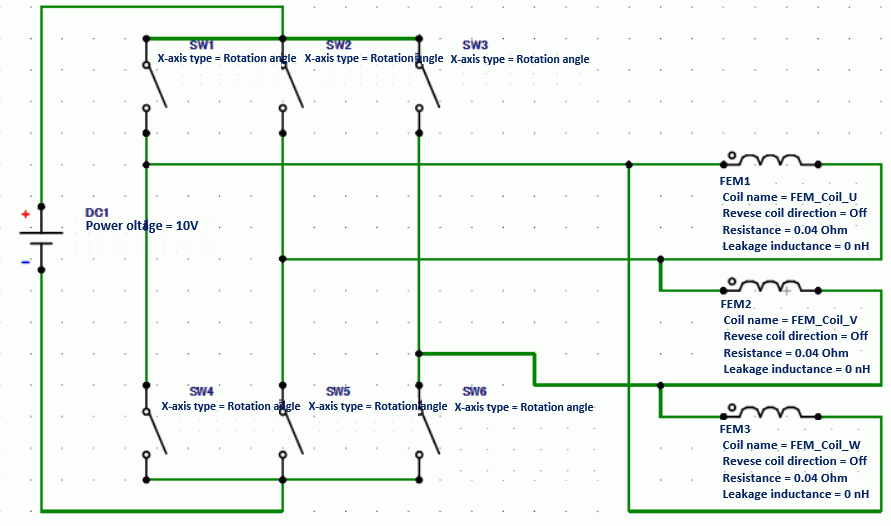Of the six switches in the three-phase bridge inverter circuit, one phase is in the high side,

another phase is in the low side, ant the rest is switched off.

The timing for switching is as follow.

 Mechanical Angle [deg] SW1 SW2 SW3 SW4 SW5 SW6 0 0 0 1 1 0 0 10 0 0 1 0 1 0 20 1 0 0 0 1 0 30 1 0 0 0 0 1 40 0 1 0 0 0 1 50 0 1 0 1 0 0 60 0 0 1 1 0 0

Set the Mesh Tab as follows.

 Tab Setting Item Setting Mesh Meshing Setup Set the general mesh size automatically: Deselect General Mesh size: 1[mm] Automatic Ambient Air Creation Select Create ambient air automatically Ambient Air Scale: 1.2

The Transient Analysis tab is set up as follows.

The number of steps is 180, circumferential division angle is 1.0[deg], and rotational quantity per step is 1[mesh]. The rotation up to 180 degrees (=180*1.0*1) is analyzed.

• As the input is by voltage for this model, it will take some time for the coil current and the torque to reach the steady state.

• To calculate the iron loss, it is necessary to set the timesteps for one period of electric angle in the steady state, and to define the iron loss characteristic for the material property.

Tab

Setting Item

Setting

Transient Analysis

Timestep

Automatic

Table

 Number Calculation steps Output steps 1 180 1

Model

A rotor core and magnet are placed in the center. A stator and coils are placed around them.

The motor has 12 poles and 18 slots.

This is a 2D model analysis.

By utilizing the symmetry, the model is 1/6 period symmetric.

Rotation period boundary (symmetric) is set.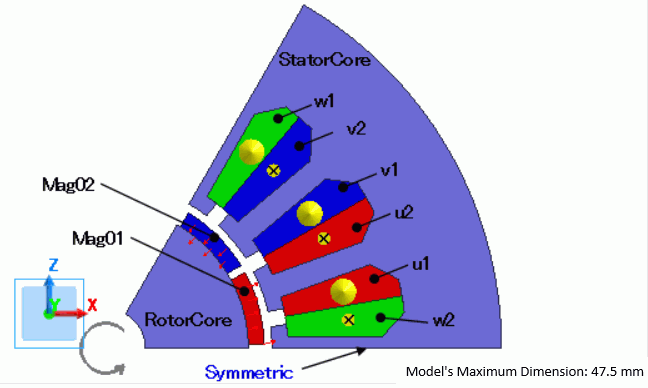Body Attributes and Materials

 Body Number/Type Body Attribute Name Material Name 4/Sheet Mag01 mag 5/Sheet Mag02 mag 0/Sheet RotorCore 50H350_Nippon Steel * 19/Sheet StatorCore 50H350_Nippon Steel * 21/Sheet u1 008_Cu * 22/Sheet u2 008_Cu * 24/Sheet v1 008_Cu * 23/Sheet v2 008_Cu * 25/Sheet w1 008_Cu * 20/Sheet w2 008_Cu *

* Available from the material DB

The body attribute is set up as follows.

For the core, the homogenizing method is selected to simulate the layered steel plates.

 Body Attribute Name Tab Setting Mag01 Direction Direction Type: Centripetal direction Coordinates of the center axis: X=0, Y=0, Z=0 Vector of the center axis: X=0, Y=1, Z=0 Outward Stator/Rotor/Air Rotor Mag02 Direction Direction Type: Centripetal direction Coordinates of the center axis: X=0, Y=0, Z=0 Vector of the center axis: X=0, Y=1, Z=0 Inward Stator/Rotor/Air Rotor RotorCore Layer Select Take layer into account Space: 97[%] Layer direction vector: X=0, Y=1, Z=0 Stator/Rotor/Air Rotor StatorCore Layer Select Take layer into account Space: 97[%] Layer direction vector: X=0, Y=1, Z=0 Stator/Rotor/Air Stator u1 Current Waveform: External circuit coupling Coil name on the circuit: FEM_Coil_U Turns: 70[Turns] Direction: – Y direction Stator/Rotor/Air Stator u2 Current Waveform: External circuit coupling Coil name on the circuit: FEM_Coil_U Turns: 70[Turns] Direction: +Y direction Stator/Rotor/Air Stator v1 Current Waveform: External circuit coupling Coil name on the circuit: FEM_Coil_V Turns: 70[Turns] Direction: – Y direction Stator/Rotor/Air Stator v2 Current Waveform: External circuit coupling Coil name on the circuit: FEM_Coil_V Turns: 70[Turns] Direction: +Y Direction Stator/Rotor/Air Stator w1 Current Waveform: External circuit coupling Coil name on the circuit: FEM_Coil_W Turns: 70[Turns] Direction: – Y direction Stator/Rotor/Air Stator w2 Current Waveform: External circuit coupling Coil name on the circuit: FEM_Coil_W Turns: 70[Turns] Direction: +Y Direction Stator/Rotor/Air Stator

The material properties are set as follows.

* [50H350_Nippon Steel] from the Material DB is used for the core.

The values for the B-H curve and the iron loss table are taken from the database. The iron loss calculation type is manually changed to [Frequency Analysis (FFT)] from [Amplitude].
Although the iron loss calculation by the iron loss table is executed by comparing the the amplitude of the frequency and the magnetic flux density with the table,
if the sine wave is not used, the harmonic component of the iron loss cannot be taken into account unless the frequency analysis (FFT) is performed.
See Permeability tab for detail.

 Material Name Tab Properties mag Permeability Material Type: Permanent magnet Magnetization Characteristic Type: Linear Magnetization Strength: 0.7[T] Relative Permeability: 1.05 50H350_Nippon Steel Iron Loss Calculation Method: Frequency Analysis (FFT) Fundamental Frequency: Calculated from the number of poles and rotations. The Number of Poles: 12

Boundary Condition

Half period symmetric boundary is set.

 Boundary Condition Name/Topology Tab Boundary Condition Type Setting Symmetric Symmetry/Continuity Periodic Rotation Period (Half Period)

Results

The below are the results.

The distribution of the magnetic flux density and magnetic flux lines at the rotation angle of 0 [deg] are shown below.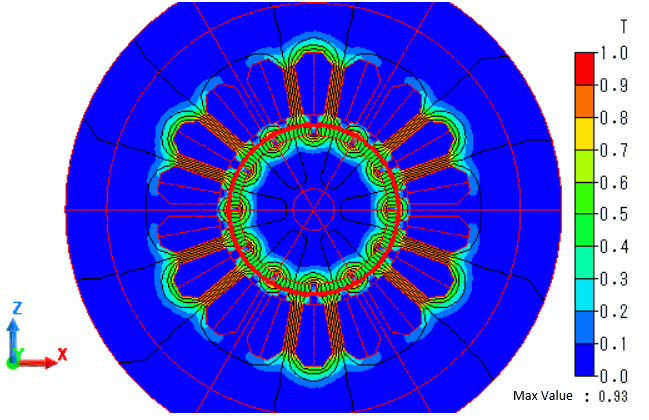The wave of torque is shown as follows. It is output to “Torque [N*m]” of the result table.

The torque reaches the steady state at 90 [deg].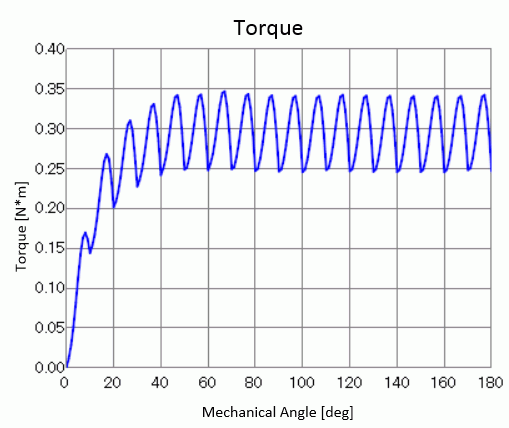The losses are listed in the table of [Loss [W] (referring to value over 1 period from the final step)].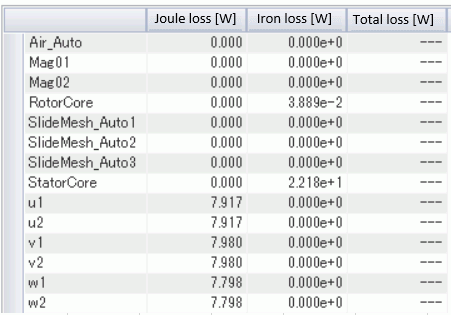﻿